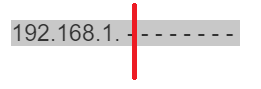In this lesson, I will explain you how you can do subnetting without the use of a subnetting calculator.

Subnetting is a difficult topic to be understood by a lot of students and engineers, that's why I will explain it in the easiest way so you can understand it once for all and I will leave for you a video showing an exercise for subnetting. Also it is ideal once you understand this topic to create a subnetting cheat sheet for your reference.

Let's start directly with the explanation. Let's say that you have this network 192,168.1.0/24 and you want to divide it as following:

2 Sub-networks and each has at least 75 hosts.

This is a requirement that you need so you can put the Finance department in a subnetwork which is different than the Sales department which is going to be in another subnetwork (let's imaging you are doing VLAN's and assign each department in a different VLANs).

Let's take the global network:
192.168.1.0/24

This means that 192.168.1 belong to the network part and .0 belong to the host part as following:

192.168.1. - - - - - - - -

So you see we have 8 bit in the last octet which is for the host, and this is defines by the subnet mask which is /24.

Now we need to check how many bits we need to borrow from this host to go to the network so we have 2 subnetworks.
To do this, we have to say 2 to the power how much will give me 2 networks? Then the answer is one.

Then we write: 21 = 2

This means that I have to borrow only 1 bit from the host to the network

Then the result will be:Let's see how many hosts we have left for each of the 2 subnetworks.

We do 27 - 2 = 128 - 2 = 126 Hosts.

Why we use 7? because those are the bits left for the hosts. And why we do -2? because from the hosts there is 1 going to the network id and one for the broadcast. I will show you about this in a moment.

So the result, we have 126 Hosts in each of the 2 subnetworks, that's more than enough. Now we can start doing the calculation.

1st we have to do the following calculation so we know what is the 1st subnetwork and what is the 2nd one:

256 - 128 = 128

We always need to take 256 because that a complete octet (28 = 256). And we subtract from it 128 because we have borrowed 1 bit from the host to the network, and this bit in decimal is 128. So now we know that we should jump 128 from one network to another.

Let me show you what the result will be then

1st Subnetwork: 192.168.1.0

2nd Subnetwork: 192.168.1.128

You see, I had to jump 128 from 1 network to another.
Now let's see what are the subnet mask, the 1st usable IP, last usable IP and broadcast ID of each subnetwork.

1st Subnetwork: 192.168.1.0
1st usable IP: 192.168.1.1
Last usable IP: 192.168.1.126

Let me explain here. We know about the Network ID. The subnet mask is 255.255.255.128 (/25) because we have borrowed 1 bit from the host to the network part, so that gives us 25 bits which is in decimal 255.255.255.128.

The 1st IP after the network id is always the usable IP address which is 192.168.1.1. The IP which is before the next subnetwork is always the broadcast IP which is 192.168.1.127. The IP which comes before the broadcast id is always the last usable IP which is 192.168.1.126. So in total, You can use the IPs from 192.168.1.1 to 192.168.1.126 with subnet mask of 255.255.255.128 for the 1st subnetwork. That's why I said that you need to take out 2 addresses when calculating the number of hosts because one goes for the Network ID and one goes to the Broadcast.

Let's check now the 2nd subnetwork. Remember you have to jump 128. So the result will be as following:

2st Subnetwork: 192.168.1.128